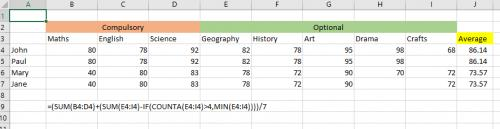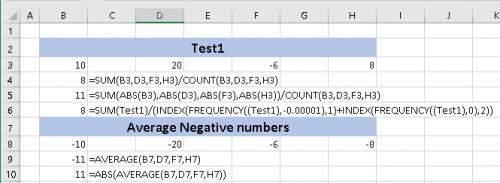Please Note: This article is written for users of the following Microsoft Excel versions: 2007, 2010, 2013, 2016, 2019, and Excel in Office 365. If you are using an earlier version (Excel 2003 or earlier), this tip may not work for you. For a version of this tip written specifically for earlier versions of Excel, click here: Averaging a Non-Contiguous Range.

# Averaging a Non-Contiguous Rangeby Allen Wyatt
(last updated October 27, 2018)

Tom needs to average a series of non-contiguous cells, excluding any cells that may contain zero values. Specifically, he needs to average cells A1, C1, E1, G1, and J1, but only counting those cells that don't contain zero.

Before getting into what works, let's take a look at what doesn't work. First of all, it doesn't work to simply add the cells and divide by 5; that doesn't take zero values into account. Second, it doesn't work to use COUNTIF in the denominator of your formula, as shown here:

```=(A1+C1+E1+G1+J1) / COUNTIF(A1:J1,"<>0")
```

This doesn't work because it examines and counts cells within the entire range of A1:J1, not just the five cells you want considered in the average. You might also think that you could select your five non-contiguous cells, give them a name, and then use the name in your formula. While Excel allows you to create the name, the following gives an error:

```=SUM(MyCells) / COUNTIF(MyCells,"<>0")
```

It appears that COUNTIF will only work with a single contiguous range, so the non-contiguous nature of the MyCells range throws the function into a tailspin. A similar problem occurs if you try to use a non-contiguous range with the AVERAGEIF function:

```=AVERAGEIF(MyCells, "<>0")
```

Since you can't use any of the functions you might want to use, you are left to rely on a bit longer formula to calculate the average. You can calculate the average of these five cells by applying a bit of "trickery" to your denominator, in this manner:

```=(A1+C1+E1+G1+J1) / ((A1<>0)+(C1<>0)+(E1<>0)+(G1<>0)+(J1<>0))
```

The evaluation done on each cell in the denominator returns either a 1 (for True) or a 0 (for False) depending on whether the cell contains a non-zero value or not. This series of values is added together, providing the necessary count of non-zero cells for the denominator.

Notice that the discussion here has been all about the denominator in the formula, not the numerator. The reason is simple—you can add all five values into the numerator; zero values there don't really matter. The only place they matter is in the denominator, which is what makes calculating this average so tricky.

ExcelTips is your source for cost-effective Microsoft Excel training. This tip (7845) applies to Microsoft Excel 2007, 2010, 2013, 2016, 2019, and Excel in Office 365. You can find a version of this tip for the older menu interface of Excel here: Averaging a Non-Contiguous Range.

##### Author Bio

Allen Wyatt

With more than 50 non-fiction books and numerous magazine articles to his credit, Allen Wyatt is an internationally recognized author. He is president of Sharon Parq Associates, a computer and publishing services company. ...

##### MORE FROM ALLEN

Formatting Partial Results of a Search

The Find and Replace capabilities of Word are, simply, quite astounding. This is particularly true when using wildcard ...

Discover More

Pay Attention to Case when Searching for ASCII Codes

Word allows you to search for specific ASCII codes in a document. If you use codes to search for alphabetic characters, ...

Discover More

Transposing Two Words

A common editing task is to transpose two adjacent words, so that their order is changed. While the task is common, there ...

Discover MoreSolve Real Business Problems Master business modeling and analysis techniques with Excel and transform data into bottom-line results. This hands-on, scenario-focused guide shows you how to use the latest Excel tools to integrate data from multiple tables. Check out Microsoft Excel 2013 Data Analysis and Business Modeling today!

##### More ExcelTips (ribbon)

Averaging the Last Numbers in a Column

Need to calculate a running average for the last twelve values in a constantly changing range of values? The formula ...

Discover More

Excluding Values from Averaging

Calculating an average of a group of numbers is easy. What if you want to exclude a couple of the numbers from the group ...

Discover More

An Average that Excludes Zero Values

Excel allows you to use functions and formulas to analyze your data. One way you can analyze your data is to use the ...

Discover More
##### Subscribe

FREE SERVICE: Get tips like this every week in ExcelTips, a free productivity newsletter. Enter your address and click "Subscribe."

View most recent newsletter.

If you would like to add an image to your comment (not an avatar, but an image to help in making the point of your comment), include the characters [{fig}] in your comment text. You’ll be prompted to upload your image when you submit the comment. Maximum image size is 6Mpixels. Images larger than 600px wide or 1000px tall will be reduced. Up to three images may be included in a comment. All images are subject to review. Commenting privileges may be curtailed if inappropriate images are posted.

What is four minus 2?

2019-06-28 02:42:54

JohnoP

Thanks SteveJez - gave me perfect platform to experiment with to find a solution.

2019-06-25 03:29:55

SteveJez

JohnoP,
Not sure how your data will be set out but maybe the following will help

=(SUM(B4:D4)+(SUM(E4:I4)-IF(COUNTA(E4:I4)>4,MIN(E4:I4))))/7 based on the layout in Fig 1. (see Figure 1 below)

the divide by 7 at the end is your number of subjects. Be careful of all of the brackets & their location if you retype.

HTHFigure 1. Example layout

2019-06-25 01:38:54

JohnoP

Brilliant! So useful. As an added challenge, I have students who do 7 subjects while others do 8. What if I need to average as you show yet I want to ignore the lowest result of those with 8 subjects? To add a further wrinkle, 3 subjects may not be ignored as they are compulsory.

2019-05-17 18:52:14

Peter Atherton

NNN

Here are a couple of solutions, one that includes Steve's solution. I'm not sure if you want the negative sign rmoved or not so both methos are here.

(see Figure 1 below)Figure 1.

2019-05-17 07:28:25

SteveJez

NNN,
Inspired by your solution, does this work for you;
I named a range of non contiguous cells - test1

=SUM(test1)/(INDEX(FREQUENCY((test1),-0.00001),1)+INDEX(FREQUENCY((test1),0),2))

it seems to do what you're describing.

HTH

2019-05-17 07:05:36

SteveJez

NNN,
Inspired by your solution, does this work for you;
I named a range of non contiguous cells - test1

=SUM(test1)/(INDEX(FREQUENCY((test1),-0.00001),1)+INDEX(FREQUENCY((test1),0),2))

it seems to do what you're describing.

HTH

2019-05-16 22:05:57

NNN

Hi there

I used below formula to get average of non contiguous cell however it doesn't work when there is negative value. how can I avoid negative

P3 cell will have -300
R3 cell has -400
U3 has -100
x3 has -50
AA3 has -50

=SUM(P3,R3,U3,X3,AA3)/INDEX(FREQUENCY((P3,R3,U3,X3,AA3),0),2)

2018-12-14 02:44:54

SteveJez

Marc,

Try the following;
=IFERROR((G21+J21+M21)/((G21<>0)+(J21<>0)+(M21<>0)),"Nothing to Average")

2018-12-13 15:30:58

Marc

Hi,
Thank you!!! Your suggestion above works great.
In my file I am averaging 3 cells, most of the time there is at least 1 or 2 cells with values, but occasionally all the cells are 0 and I get the following #DIV/O!. Is there away to fix this or ignoring the average calculation when all are zero..?

=(G21+J21+M21)/((G21<>0)+(J21<>0)+(M21<>0))

Really appreciate your assistance
Thank you,
Marcus

{fig}

2018-10-27 16:35:01

Eric J

This will also do the trick.

=SUM(MyCells)/(COUNT(MyCells)-FREQUENCY(MyCells,0))

Frequency will count the (0) zeros in the MyCells array and ignore blank cells and text.
Count will tally the cells in the array with numbers only.

##### This Site

Got a version of Excel that uses the ribbon interface (Excel 2007 or later)? This site is for you! If you use an earlier version of Excel, visit our ExcelTips site focusing on the menu interface.